# Problems of the Week

Contribute a problem

# 2017-08-21 Intermediate

True or False?

It is possible to partition the set of the first 2026 positive integers $\{1, 2, 3, \dots, 2026\}$ into exactly two subsets such that the product of all the elements in each subset is the same.


Note: 2027 is a prime number.

A simple pendulum consists of a small object dangling from a piece of string. A creative student constructs 8 pendulums of different lengths: $5^2 \text{ cm},\ 6^2 \text{ cm},\ 7^2 \text{ cm},\ ...,\ 11^2 \text{ cm},\ 12^2 \text{ cm},$ and hangs them next to each other in increasing order as shown in the diagram below. Thus, $L_n = \SI{(n+4)^2}{cm}$: the shortest pendulum has length $L_1 = \SI{25}{cm},$ and the longest $L_8 = \SI{144}{cm}$.

The student now starts the pendulums, by pulling them all sideways over the same short distance and releasing them at the same time $t = 0$. Because of their different lengths, the pendulums swing at different frequencies and will soon all be completely out of phase.

How long will it take for all these pendulums to be exactly in phase again?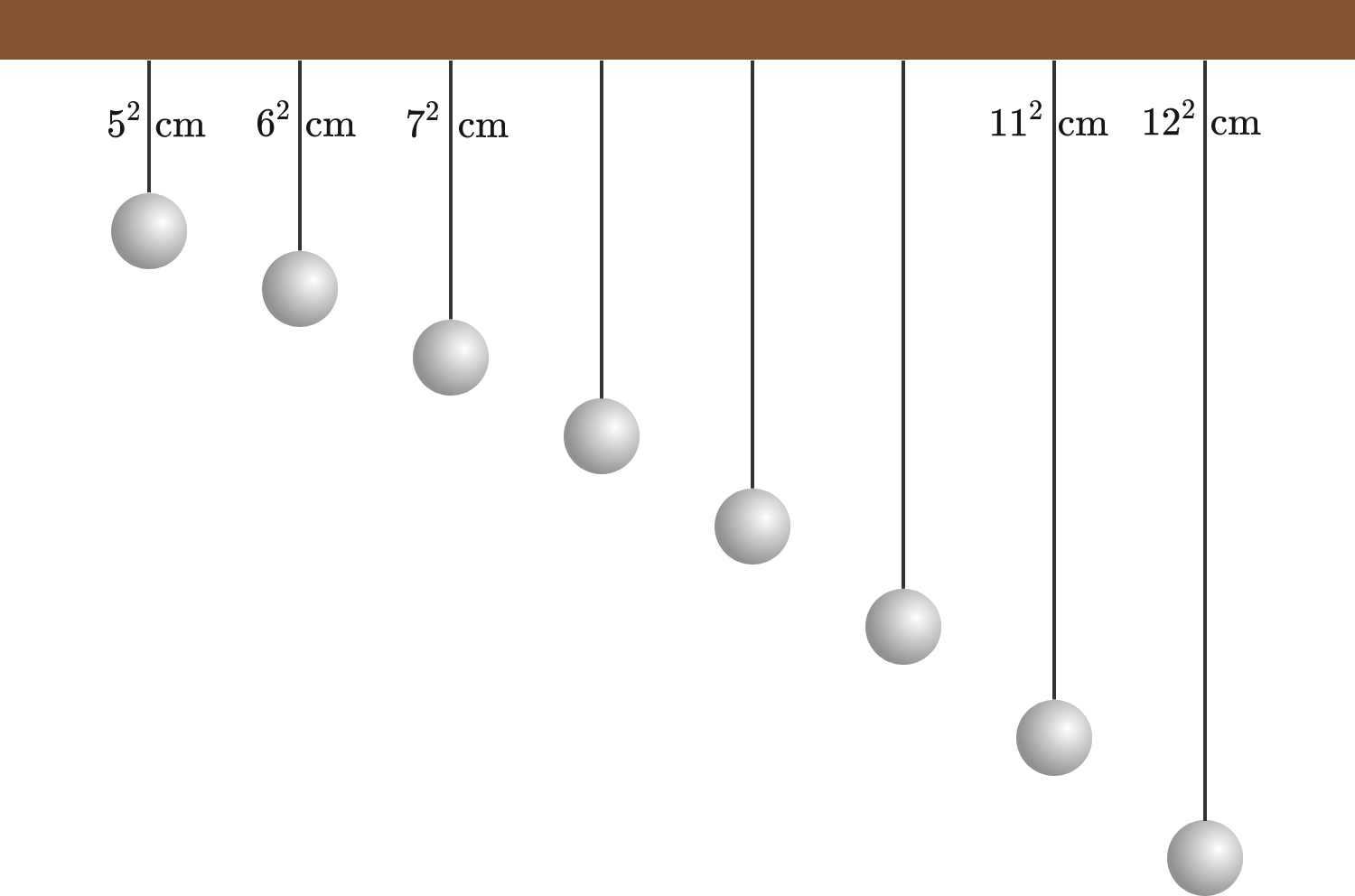Details and Assumptions:

• $g = \SI{9.81}{m/s^2}.$
• The pendulums are ideal, simple pendulums with period $T_n = 2\pi\sqrt{\frac {L_n}g}.$
• For a demonstration of this kind of setup, see this video.

A queen bee has two parents: one male and one female.
A male bee has just one parent, a queen bee.

If we go back just one generation, a queen bee has two ancestors.
If we go back two generations, this rises to three ancestors in that generation.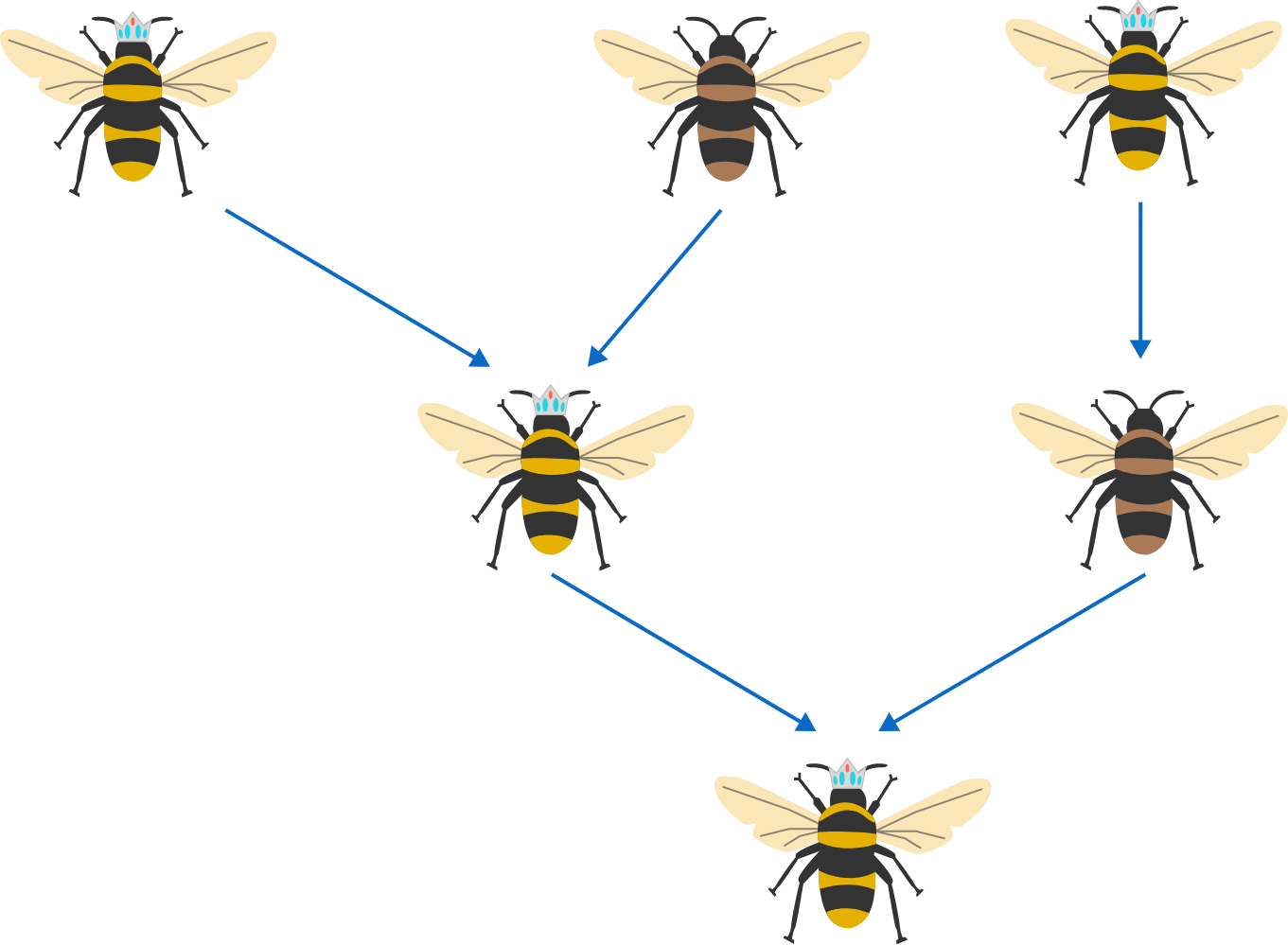How many ancestors does a queen bee have going back 20 generations (again, only in that generation)?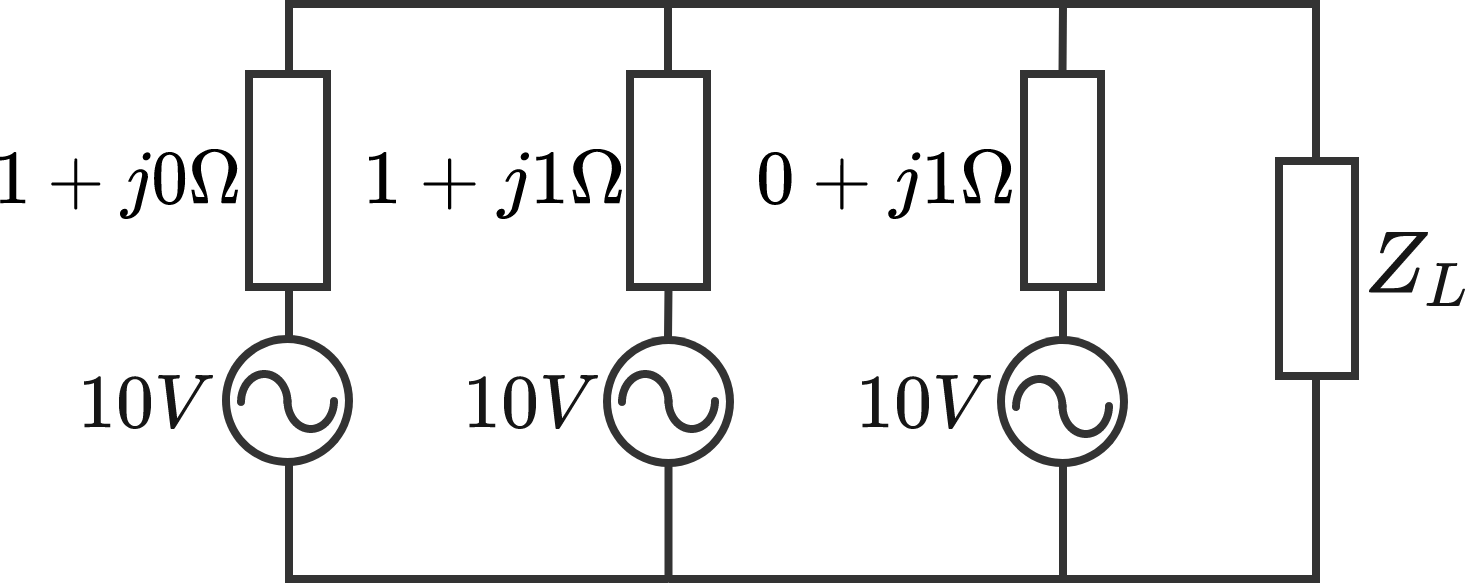Consider the AC circuit shown above, with source impedances and RMS source voltages given. The load impedance $Z_L$ internally consists of a combination of resistors, capacitors, and inductors.

What is the maximum active power (in watts) which can possibly be dissipated by the load?

There are nine $3 \times 3$ sub-grids in the $5 \times 5$ grid. If at most 5 out of 9 unit squares are shaded blue in any $3 \times 3$ sub-grid, what is the maximum number of unit squares in the $5 \times 5$ grid that can be shaded blue?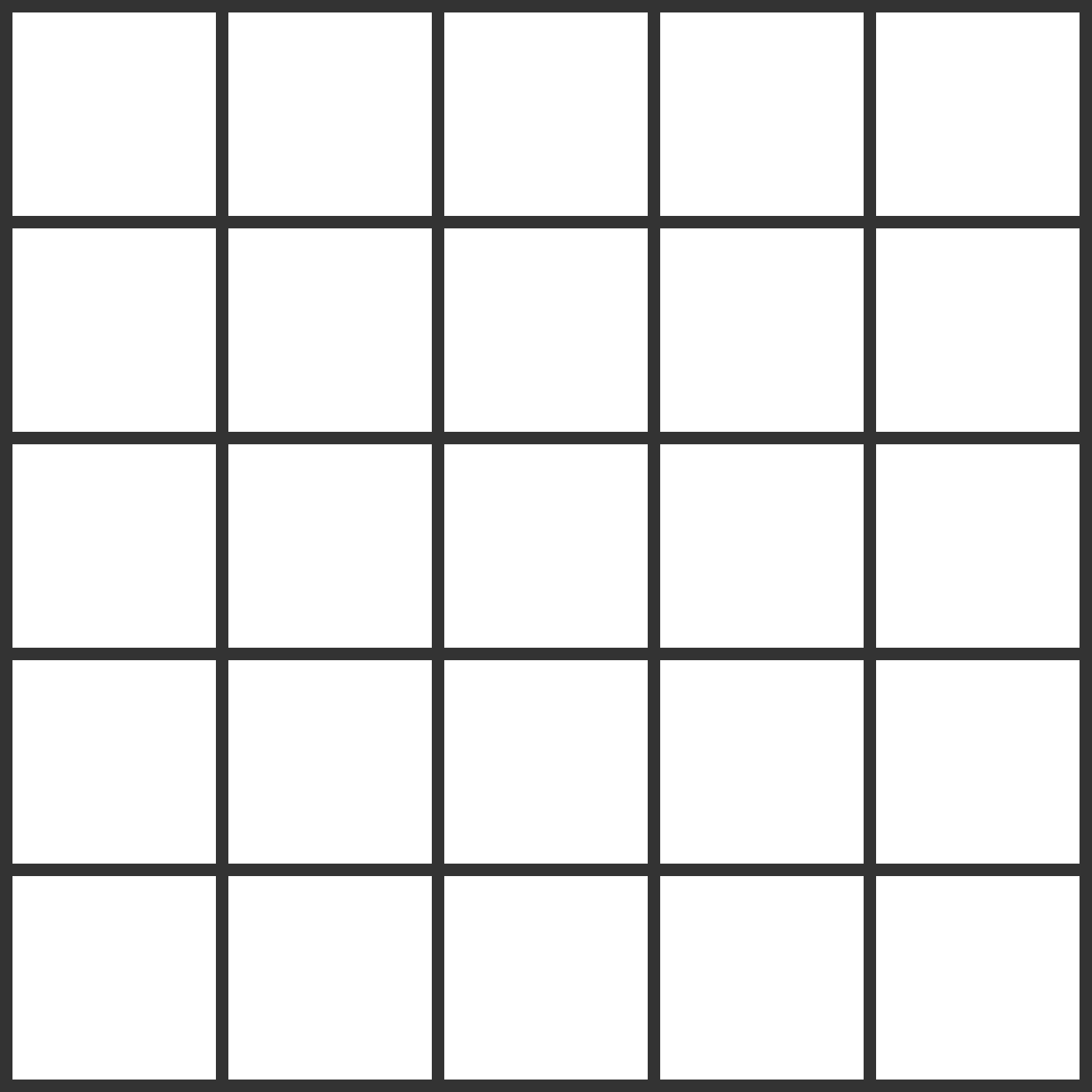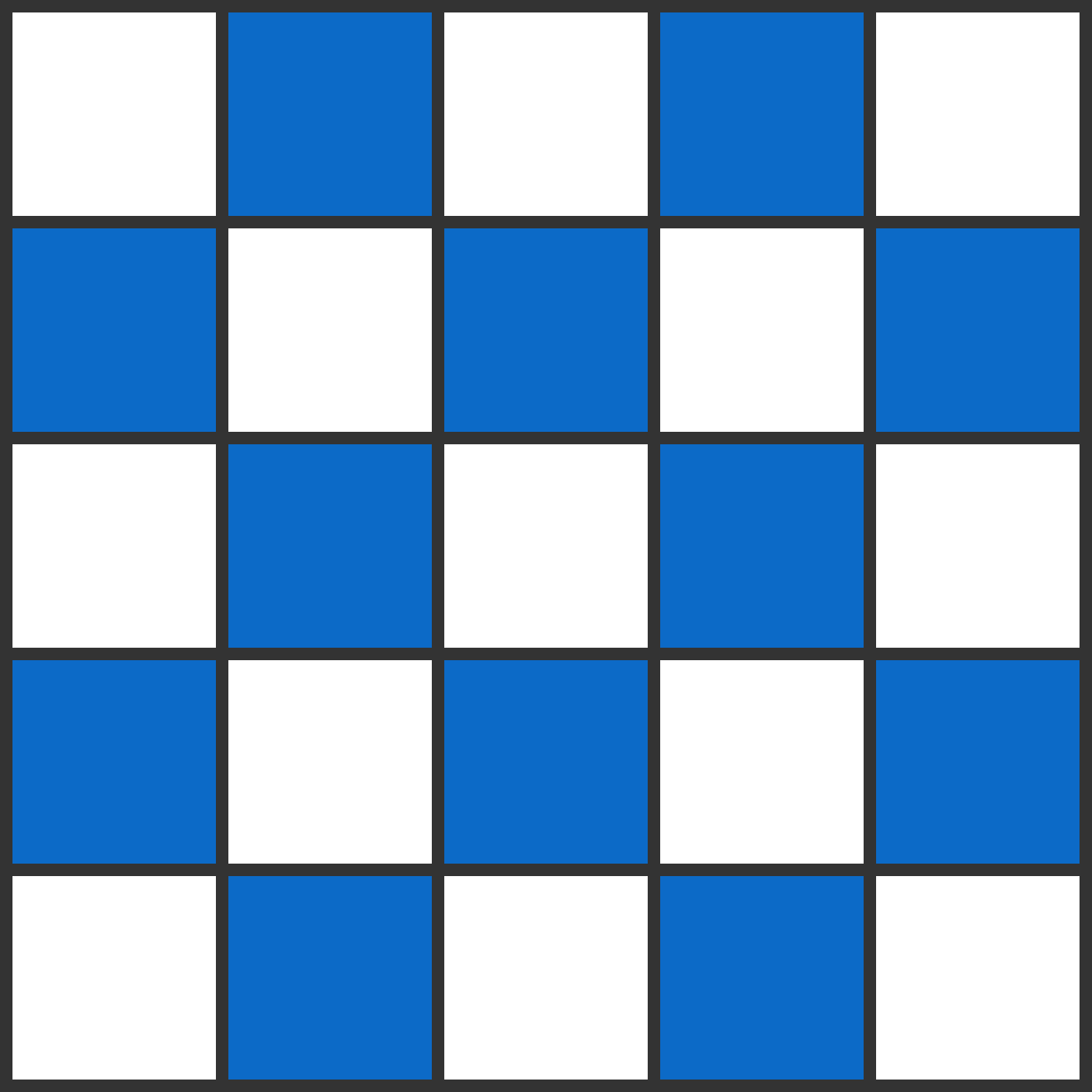Note: An example of how the grid can be shaded is shown to the right.

×